##### Managerial Economics For Dummies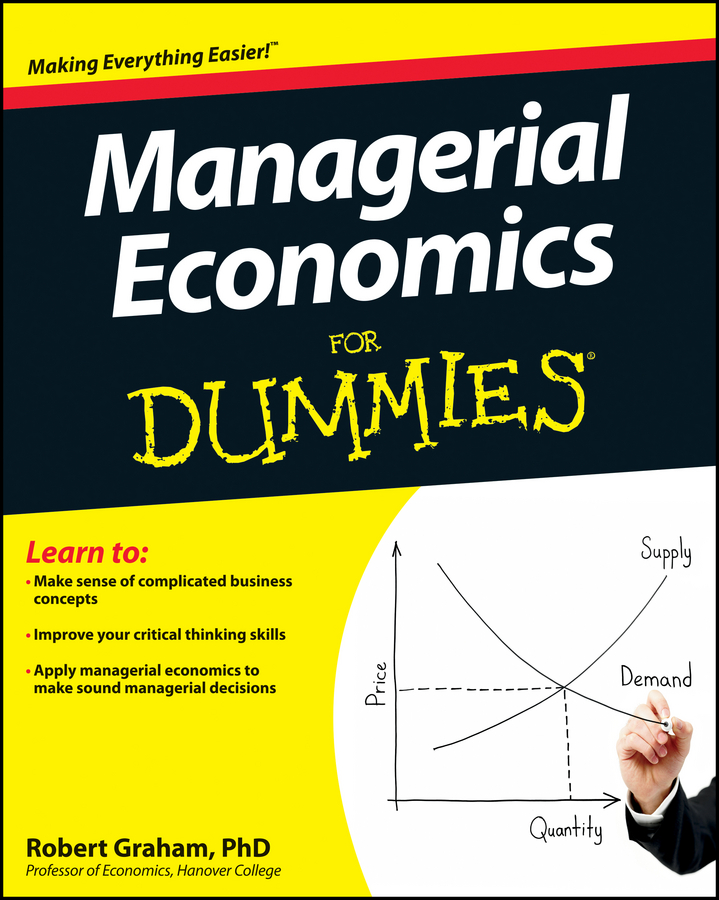In order to determine the profit-maximizing price and quantity for each group of customers by using third-degree price discrimination, you must satisfy the following condition: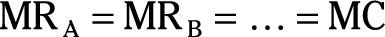where MRA is group A’s marginal revenue for the last unit it buys, MRB is group B’s marginal revenue for the last unit it buys, and MC is marginal cost for the last unit you produce.

Assume that the following equations describe the demand for your firm’s product for two different groups of customers. Group A’s demand is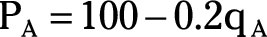where PA is the price in dollars charged to group A customers, and qA is the quantity sold to group A customers. Group B’s demand is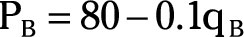where PB is the price in dollars charged to group B customers, and qB is the quantity sold to group B customers.

Your firm’s total cost and marginal cost equations arewhere TC is your total cost in dollars, MC is marginal cost in dollars, and q is the total quantity of the good your firm produces.

Using this information and the following steps, you can determine the price to charge each group of consumers, how much to sell each group of consumers, and how much to produce:

1. Determine the marginal revenue for group A customers.

First, multiply the demand equation by qA to determine total revenue, and then take the derivative of total revenue with respect to qA to determine marginal revenue.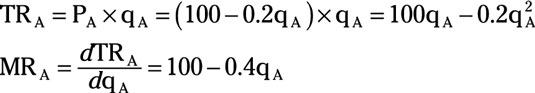2. Determine the marginal revenue for group B customers.

First, determine total revenue by multiplying PB by qB, and then take the derivative of total revenue with respect to qB to determine marginal revenue.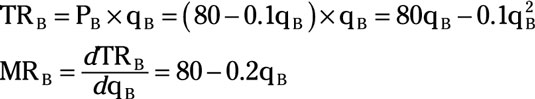3. Set MRA = MC.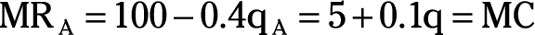4. Substitute qA + qB for q.

The total quantity of output you produce and sell, q, is sold to customers in either group A or group B.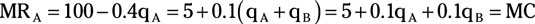5. Solve the equation in Step 4 for qB.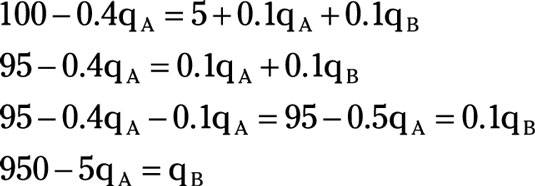6. Set MRA equal to MRB.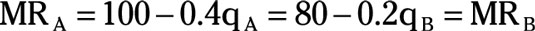7. Substitute qB = 950 – 5qA in the equation in Step 6.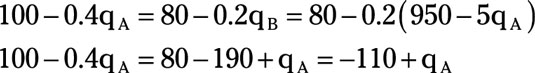8. Solve for qA.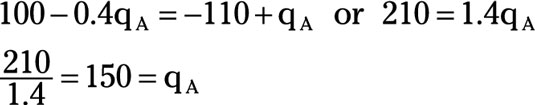9. Substitute qA = 150 in group A’s demand equation to determine the price, PA, to charge customers in group A.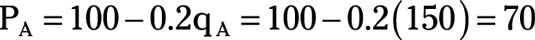10. Substitute qA = 150 in the equation from Step 5 to determine qB.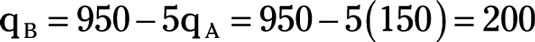11. Substitute qB = 200 in group B’s demand equation to determine the price, PB, to charge customers in group B.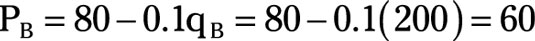12. Determine the total quantity of output that your firm produces.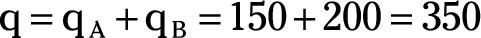Your firm produces 350 units of the good. It sells 150 units to customers in group A at a price of \$70 per unit and 200 units to customers in group B at a price of \$60 per unit.